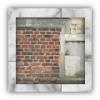#### You may also like### Rule of Three

If it takes four men one day to build a wall, how long does it take 60,000 men to build a similar wall?### Crossing the Atlantic

Every day at noon a boat leaves Le Havre for New York while another boat leaves New York for Le Havre. The ocean crossing takes seven days. How many boats will each boat cross during their journey?### Fixing the Odds

You have two bags, four red balls and four white balls. You must put all the balls in the bags although you are allowed to have one bag empty. How should you distribute the balls between the two bags so as to make the probability of choosing a red ball as small as possible and what will the probability be in that case?

# Designing Table Mats

### Why do this problem?

This problem provides a context from DT (designing an object for marketing) for formulating a mathematical model and investigating it.  The investigation could be done by hand (as in the solution), or using a spreadsheet.  More advanced students could be encouraged to use an algebraic approach.

### Possible approach

A good place to start is by drawing the coils of rope on square paper.  Doing this should lead to discussion about assumptions which need to be made, such as:

• a suitable diameter for the rope and length for the initial section of rope
• coils fit together without any gaps
• all sections of the coils are either horizontal or vertical

The drawing can then be used to investigate how the dimensions of the finished mat increase as additional sections or coils of rope are added - learners will need to decide whether they want to focus on the number of coils as each new rectangle is formed, or the number of sections of rope.

### Key questions

• How does the length of the initial section of rope affect what finished dimensions are possible?
• How do the length and width increase with the number of coils or sections?
• Revisiting the initial assumptions, which do we think are reasonable, which are less so?  How much do they affect our calculations?

### Possible extension

Compare the analysis of rectangular mats with a circular mat constructed in a similar way.  To compare which design is more economical requires finding the length of rope needed, to see which requires the least.  This could be done by argument - that a circular mat of a given diameter will require less rope than a square mat of the same length.  Alternatively the circular mat could be modelled as a series of concentric circles.

### Possible support

Have rope or thick string available for learners to experiment with.